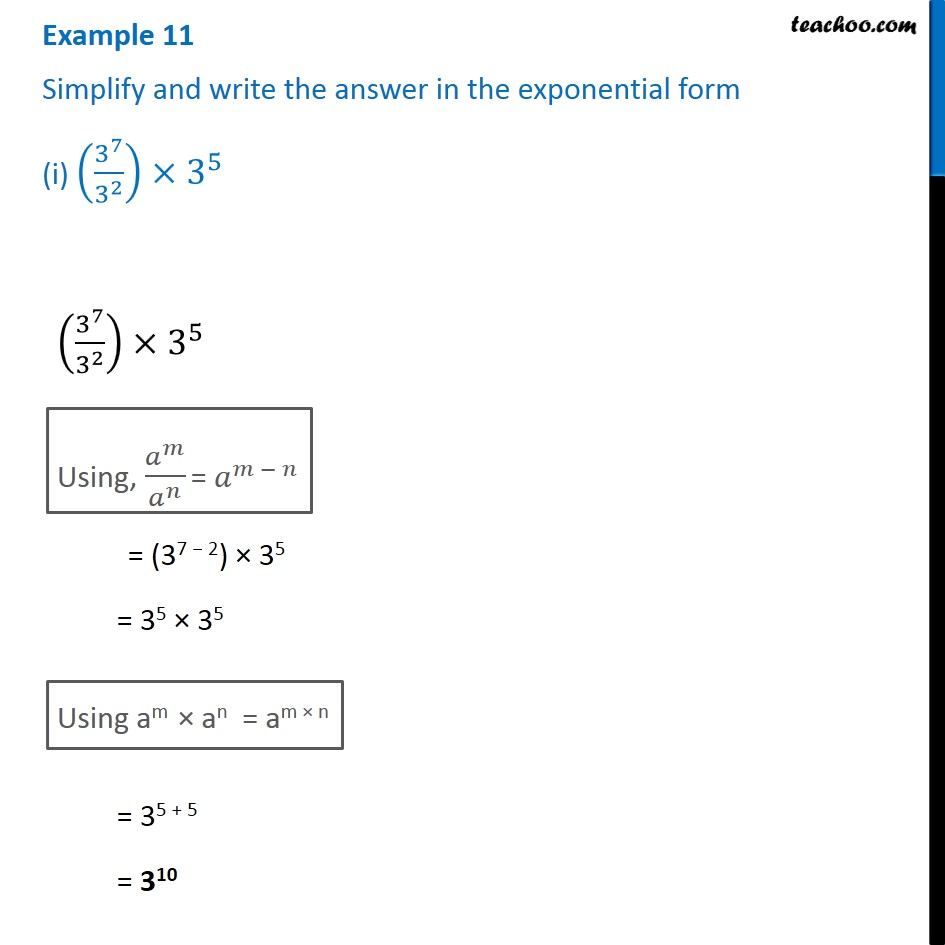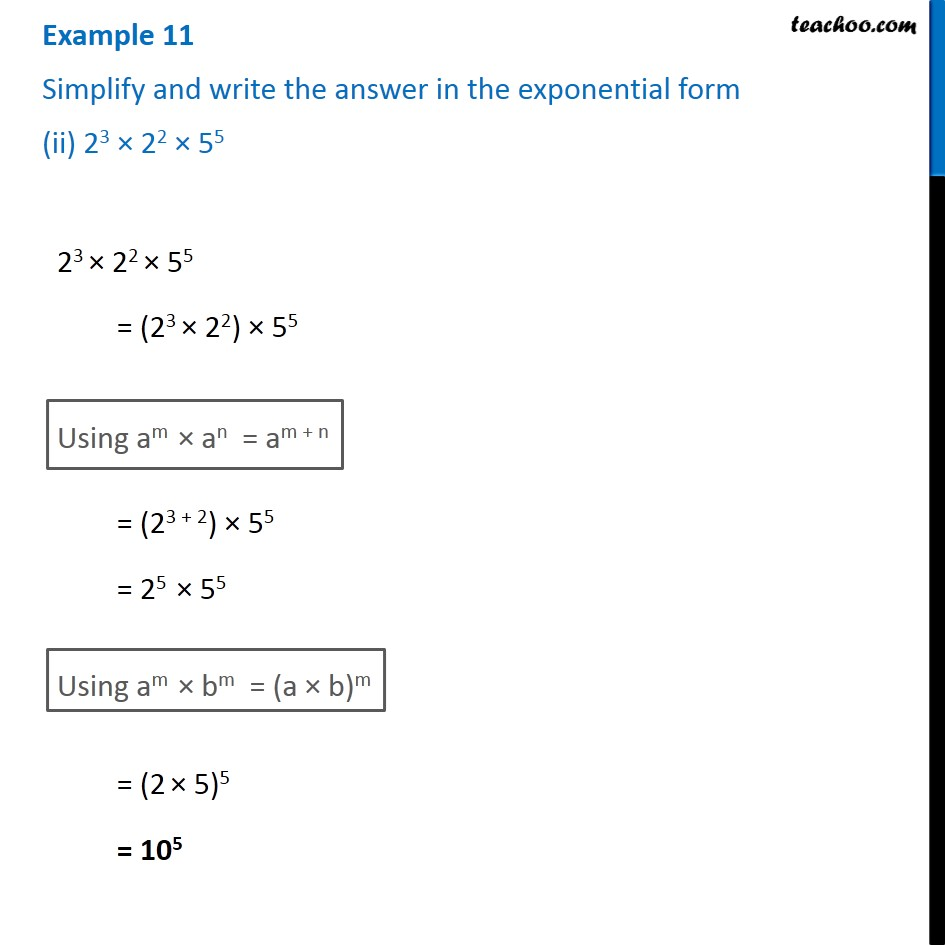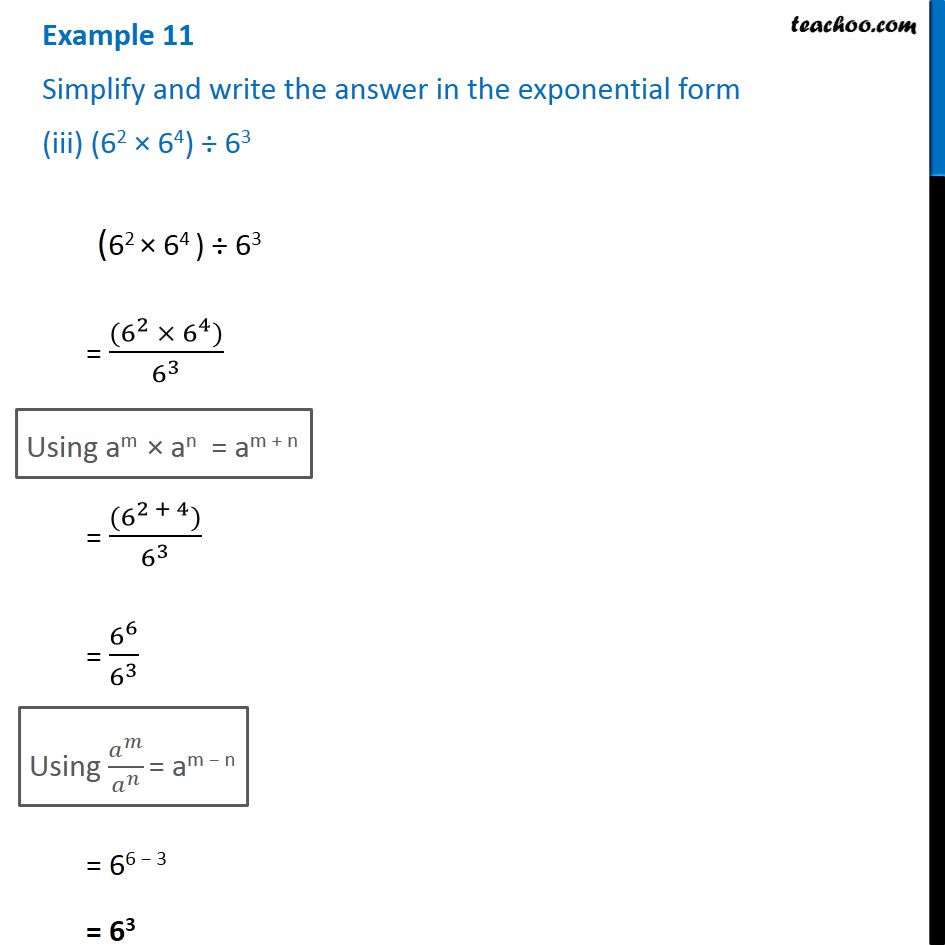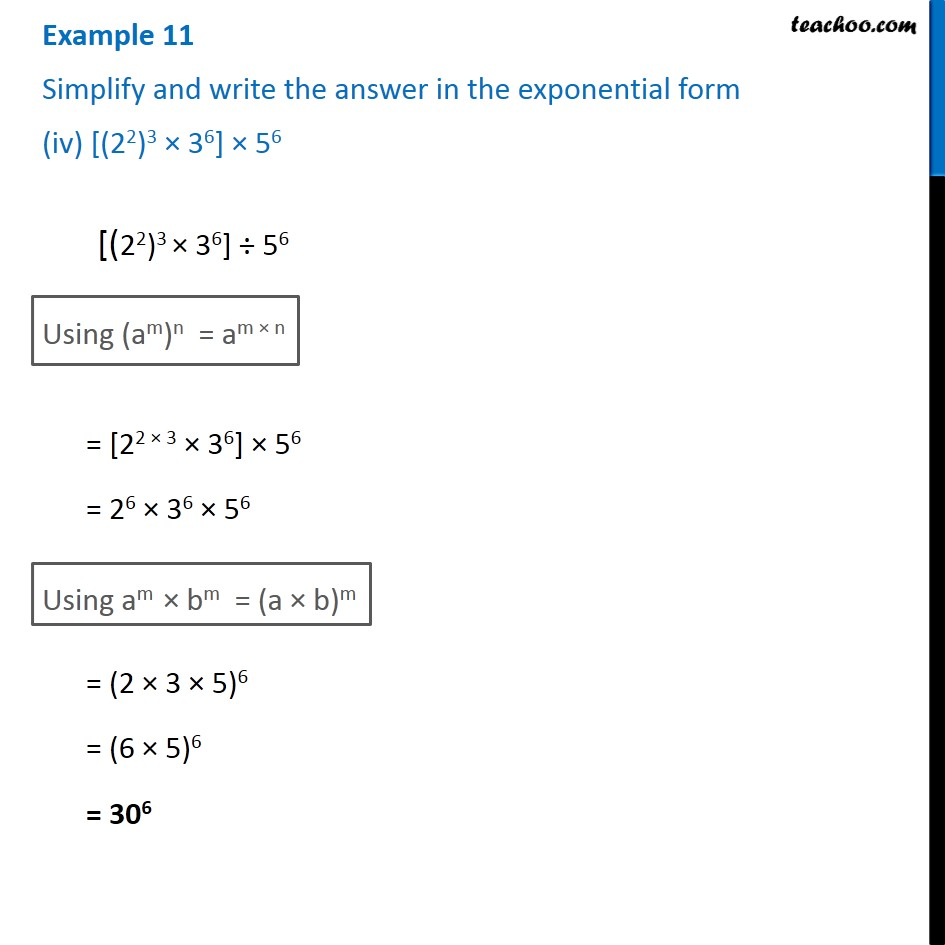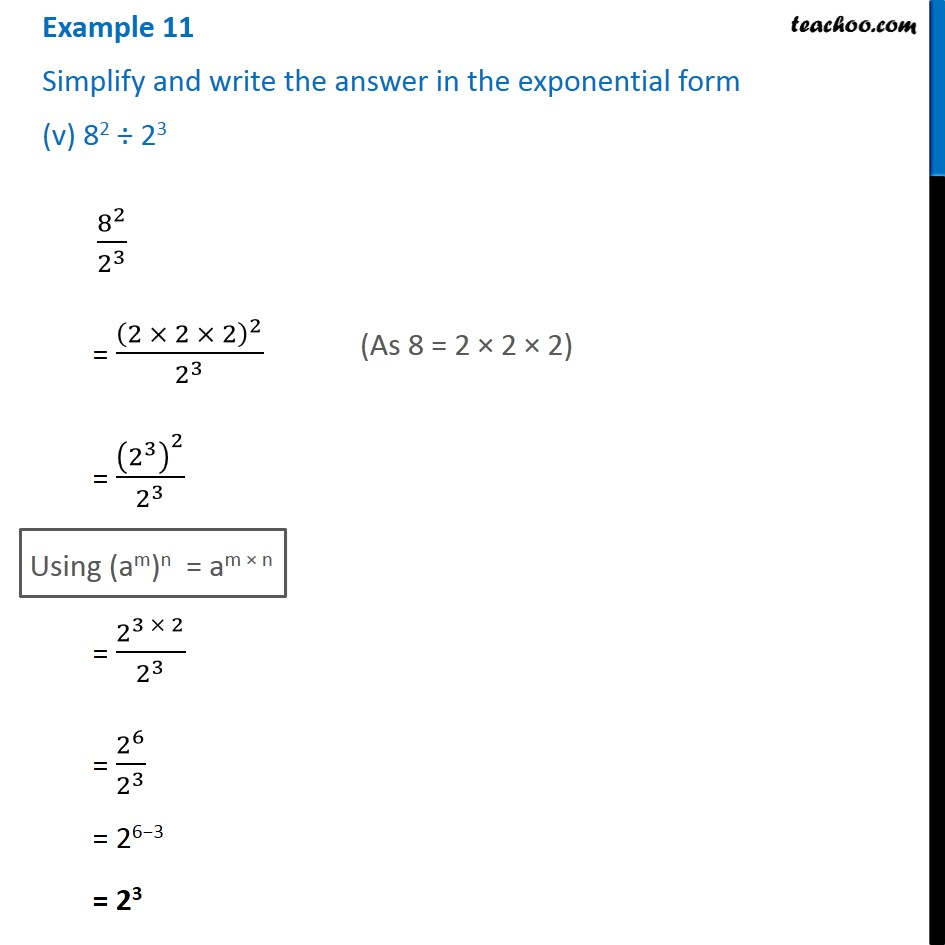1. Chapter 13 Class 7 Exponents and Powers
2. Serial order wise
3. Examples

Transcript

Example 11 Simplify and write the answer in the exponential form (i) (3^7/3^2 )×3^5 (3^7/3^2 )×3^5 Using, 𝑎^𝑚/𝑎^𝑛 = 𝑎^(𝑚 − 𝑛) = (37 − 2) × 35 = 35 × 35 Using am × an = am × n = 35 + 5 = 310 Example 11 Simplify and write the answer in the exponential form (ii) 23 × 22 × 55 23 × 22 × 55 = (23 × 22) × 55 Using am × an = am + n = (23 + 2) × 55 = 25 × 55 Using am × bm = (a × b)m = (2 × 5)5 = 105 Example 11 Simplify and write the answer in the exponential form (iii) (62 × 64) ÷ 63 (62 × 64 ) ÷ 63 = ((6^2 ×〖 6〗^4))/6^3 Using am × an = am + n = ((6^(2 + 4)))/6^3 = 6^6/6^3 Using 𝑎^𝑚/𝑎^𝑛 = am − n = 66 − 3 = 63 Example 11 Simplify and write the answer in the exponential form (iv) [(22)3 × 36] × 56 [(22)3 × 36] ÷ 56 Using (am)n = am × n = [22 × 3 × 36] × 56 = 26 × 36 × 56 Using am × bm = (a × b)m = (2 × 3 × 5)6 = (6 × 5)6 = 306 Example 11 Simplify and write the answer in the exponential form (v) 82 ÷ 23 8^2/2^3 = (2 × 2 × 2)^2/2^3 = (2^3 )^2/2^3 (As 8 = 2 × 2 × 2) Using (am)n = am × n = 2^(3 × 2)/2^3 = 2^6/2^3 = 26−3 = 23

Examples